Next: 9. Theory Interpretations Up: 2. User's Guide Previous: 7. Logic Overview

Subsections

# 8.1 Introduction

As a mathematical object, an IMPS theory consists of a LUTINS language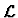and a set of sentences incalled axioms. The theory is the basic unit of mathematical knowledge in IMPS. In fact, IMPS can be described as a system for developing, exploring, and relating theories. How a theory is developed is the subject of several chapters; an overview is given in the next section. The principal technique for exploring a theory is to discover the logical implications of its axioms by stating and trying to prove conjectures; the IMPS proof system is described in Chapter 12. Two theories are related by creating an interpretation of one in the other; theory interpretations are discussed in Chapter 9.

We define a theorem of an IMPS theory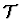to be a formula ofwhich is valid in each model for. That is, a theorem ofis a semantic consequence of. The reader should note that our definition of a theorem does not depend on the IMPS proof system or any other proof system for LUTINS.

In the implementation, a theory is represented by a data structure which encodes the language and axioms of the theory. The data structure also encodes in procedural or tabular form certain consequences of the theory's axioms. This additional information facilitates various kinds of low-level reasoning within theories that are encapsulated in the IMPS expression simplifier, the subject of Chapter 13.

Let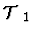and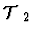be IMPS theories.is subtheory of(andis a supertheory of) if the language ofis a sublanguage of the language ofand each axiom ofis a theorem of. Each IMPS theory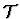# 8.2 How to Develop a Theory

The user creates a theory and the IMPS objects associated with it by evaluating expressions called definition forms (or def-forms for short). There are approximately 30 kinds of def-forms, each described in detail in Chapter 17. The user interface provides templates to you for writing def-forms. The def-forms supplied by the system and created by you are stored in files which can be loaded as needed into a running IMPS process. In this section, we give an overview of the tasks that are involved in creating a well-developed theory. Along the way, we list the def-forms that are relevant to each task.

## Task 1: The Primitive Theory

The first task in developing a theory is to build a primitive, bare bones theoryThe information held in the axioms of the primitive theory is unlocked by proving theorems using the IMPS proof system (see Chapter 12). Theorem proving is crucial to developing a theory and is involved in most of the tasks below. Proven theorems are installed in the theory with def-theorem using various usages.'' See Section 8.4 for details.

Once the primitive theory is built, usually the next task is to specify how simplification should be performed in the theory. There are three mechanisms for doing this that can be used separately or in combination:

1.
Install a processor. For theories of an algebraic nature (e.g., rings, fields, or modules over a ring), you may want to install an algebraic processor to perform rudimentary algebraic simplification. Processors are created with def-algebraic-processor and def-order-processor, and installed with def-theory-processors. The system will check that the theory contains the right theorems before anything is installed, so even if you build a weird processor (one that wants to treat ring multiplication as ring addition) it cannot be installed.

2.
Install rewrite rules. An ordinary rewrite rule is created and installed in the theory by installing a theorem with the usage rewrite. A transportable rewrite rule is created by installing a theorem with the usage transportable-rewrite, and is installed in the theory with def-imported-rewrite-rules.

3.
Add information to the theory's domain-range handler. Information about the domain and range of function constants can be added to a theory by installing theorems in the theory with the usages d-r-convergence and d-r-value.

See Chapter 13 for more details.

It is a good idea, early in the development of a theory, to create the principal theory interpretations involving the theory in either the role of source or target. Symmetry interpretations of theory in itself are often quite useful if they exist. Theory interpretations are created with def-translation. See Chapter 9 for details.

Atomic sorts and constants can be defined throughout the course of developing a theory. They are usually defined with def-atomic-sort, def-constant, and def-recursive-constant, but they can also be created by transporting definitions to the theory from some other theory with def-transported-symbols. An atomic sort with constants for representing a cartesian product with a constructor and accessors is created with def-cartesian-product. See Section 8.5 for details.

Theorems are applied semi-automatically in the course of a proof by means of procedures called macetes. A set of carefully crafted macetes is an essential part of a well-developed theory. An elementary macete is created whenever a theorem is installed, and a transportable macete is created by installing a theorem with the usage transportable-macete. Other kinds of macetes are created with def-compound-macete and def-schematic-macete. See Chapter 14 for details.

If your theory has a theorem which you think can be treated like an induction principle, then you can build an inductor with def-inductor. The name of the inductor can be used as an argument to the induction command. Note that most often you can get by with using integer-inductor which is already supplied with the system.

Sometimes copies and unions of copies of the theory are needed. These can be created and managed as an IMPS theory ensemble with def-theory-ensemble, def-theory-ensemble-instances, def-theory-ensemble-overloadings, and def-theory-ensemble-multiple. See Chapter 11 for details.

Ordinarily an extension of the theory is created with def-theory and def-theory-instance, but the theory can also be extended by adding an abstract data type with def-bnf or a record with def-record-theory.

Quasi-constructors are global constants that can be used across a large class of theories. They are created with def-quasi-constructor. See Chapter 10 for details.

Renaming functions are used with def-theory-instance and def-transported-symbols to rename atomic sorts and constants. They are created with def-renamer.

Special parse and print syntax for the vocabulary of the theory is created independently from the development of the theory with def-parse-syntax, def-print-syntax, and def-overloading. See Chapter 16 for details.

Theory library sections containing the theory or a part of the theory are created with def-section. See Section 8.6 for more information about sections.

# 8.3 Languages

An IMPS language is a representation of a LUTINS language. An IMPS language is built with def-language from a (possibly empty) set of languages, a set of base types, and a list of atomic sort declarations.

# 8.4 Theorems

Mathematically, a theorem of a theory is any logical consequence of the theory's axioms. One usually verifies that a formula A is a theorem of a theory# 8.5 Definitions

IMPS supports four kinds of definitions: atomic sort definitions, constant definitions, recursive function definitions, and recursive predicate definitions. In the following let# 8.6 Theory Libraries

A theory library is a collection of theories, theory constituents (definitions, theorems, proofs, etc.), and theory interpretations that serves as a database of mathematics. A theory library is composed of sections; each section is a particular body of knowledge that is stored in a set of files consisting of def-forms. A section can be loaded as needed into a running IMPS process. A section is built from a (possibly empty) set of subsections and files with def-section. The forms load-section and include-files will load a section and a list of files, respectively. These forms are useful for organizing sections.

Supplied with IMPS is an initial theory library which contains a variety of basic mathematics. In no sense is the initial theory library intended to be complete. We expect it to be extended by IMPS users. In the course of using IMPS, you should build your own theory library on top of the IMPS initial theory library. The initial theory library contains many examples that you can imitate. An outline of the initial theory library is given in Chapter 20.

# 8.7 Hints and Cautions

1.
The IMPS initial theory library contains no arithmetic theory weaker than h-o-real-arithmetic; e.g., there is no theory of Peano arithmetic. This is in accordance with our philosophy that there is rarely any benefit in building a theory with an impoverished arithmetic. Furthermore, since full-powered arithmetic is so useful and so basic, we advise the IMPS user to include h-o-real-arithmetic in every theory he or she builds.

2.
When building a theory, it is usually a good idea to minimize the primitive constants and axioms of the theory. This will make it easier to use the theory as a source of a theory interpretation.

3.
The best way to learn how to develop a theory is to study some of the theory-building techniques and styles which are demonstrated in the IMPS initial theory library.

4.
Since the sort of an expression gives immediate information about the value of the expression, it is often very advantageous to define new atomic sorts rather than work directly with unary predicates. Also, a nonnormal translation (see Section 9.2) can be normalized,'' i.e., transformed into a normal translation, by defining new atomic sorts in the target theory of the translation.Next: 9. Theory Interpretations Up: 2. User's Guide Previous: 7. Logic Overview